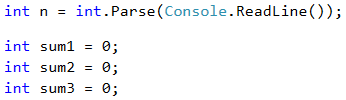# Problem: Sums with Step of 3

The next sample exam problem is about calculating 3 sums, holding the numbers from given sequence, staying at certain positions with step 3.

## Video: Sums with Step of 3

Watch the video lesson about solving the "Sums with Step of 3" problem: https://youtu.be/bRHFuNNBmZc.

## Problem Description

We are given n integers: a1, a2, …, an. Calculate the sums:

• sum1 = a1 + a4 + a7 + … (the numbers are summed, starting from the first one with step of 3).
• sum2 = a2 + a5 + a8 + … (the numbers are summed, starting from the second one with step of 3).
• sum3 = a3 + a6 + a9 + … (the numbers are summed, starting from the third one with step of 3).

## Input

The input data is read from the console. The first line holds an integer n (0 ≤ n ≤ 1000). On the next n lines we are given n integers in the range [-1000 … 1000]: a1, a2, …, an.

## Output

On the console we should print 3 lines containing the 3 sums in a format such as in the example.

## Sample Input and Output

Input Output Input Output Input Output
2
3
5
sum1 = 3
sum2 = 5
sum3 = 0
4
7
-2
6
12
sum1 = 19
sum2 = -2
sum3 = 6
5
3
5
2
7
8
sum1 = 10
sum2 = 13
sum3 = 2

We will take the count of numbers from the console and declare starting values of the three sums.Since we do not know in advance how many numbers we will process, we will take them one at a time in a loop which will be repeated n times and we will process them in the body of the loop.## Allocating Numbers and Printing Results

To find out in which of the three sums we need to add the number, we will divide its sequence number into three and we will use the remainder. We will use the variable i, which tracks the number of runs of the loop, in order to find out which sequence number we are at. When the remainder of i/3 is zero, it means we will add this number to the first sum, when it is 1 to the second one, and when it is 2 to the third one.Finally, we will print the result on the console in the required format.